## Application of Laplace Transform:

Application of Laplace Transform methods are used to find out transient currents in circuits containing energy storage elements. To find these currents, first the differential equations are formed by applying Kirchhoff’s laws to the circuit, then these differential equations can be easily solved by using Laplace transformation methods.

Consider a series RL circuit shown in Fig. 13.3.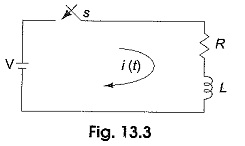When the switch is closed at t = 0, the voltage V is applied to the circuit.

By applying Kirchhoff’s laws, we get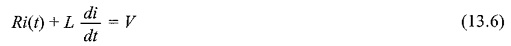Now, application of Laplace transform to each term gives,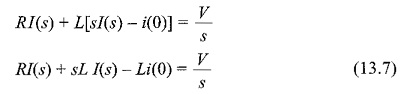i(0) is the current passing through the circuit just before the switch is closed. When i(0) = 0, Eq. 13.7, becomes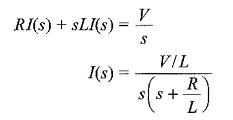The current i(t) can be determined by taking inverse Laplace transform.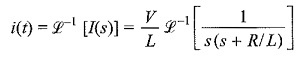To find the constants, let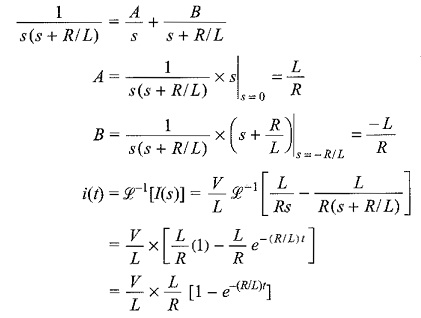Current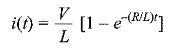Scroll to Top